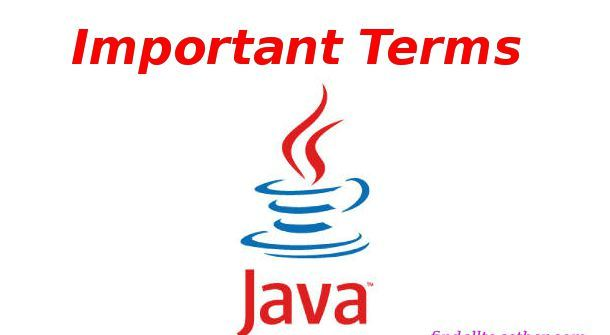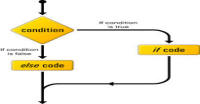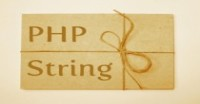# Variables and data types in Java

Variable is a memory location which stores data. In Java, we have to define what type of variable (integer, text, string, etc.), we are storing. After it a fixed memory is assign to each variable. For example, normal integer is assign 4 bytes. We can not assign other type of values to defined variables. For example, if we have defined a variable as integer then we can not assign a text string to that variable. We can modify that variable with different value of same type (say another integer). In java, there are 8 types of primitive variables.

1. Integer
2. Byte
3. Char
4. Boolean
5. Double
6. short
7. long
8. float

NOTE:

1. Run below examples by writing in main method. To print them in console use System.print.out("variable");
2. We can give variables any name other than reserved keywords starting with letter, \$ or underscore (_).

### Integer:

Integer is a 32-bit data type which stores integer values from range - 2,147,483,648 ( -2^31 ) to 2,147,483,647(inclusive) ( 2^31 -1 ).

Example:

```int a = 8;
a = 10;
```

### Example:

```byte a = 23;
a = -24;
```

### Char:

It is 16-bit unicode character which stores any character.

Example:

```char a = 'a';
a = 'z';
```

NOTE: Character is written inside single colons '' but string is written in double colons "".

### Boolean:

It is one bit boolean data type which has only two values: true or false.
Example:

```boolean a = true;
a = false;
```

### Double:

Double data type is a double-precision 64-bit IEEE 754 floating point. This should not be used for precise values such as currency.
Example:

```double a = 56;
a = 45.98;
```

### Short:

Short data type is a 16-bit signed two's complement integer. Its range is from -32,768 to 32,767 (inclusive).

### Long:

Long data type is a 64-bit signed two's complement integer. Its range is -9,223,372,036,854,775,808 to 9,223,372,036,854,775,807 (inclusive). It is used when we need wider range for integers.
Example:

```int a = 200000L;
a = -300000L;
```

### Float:

Float data type is a single-precision 32-bit IEEE 754 floating point. Float data type is also never used for precise values such as currency.

### More types:

String:

String is an array of characters. We will read about array in detail later. At this moment you may assume string is a collection of characters. It is simple text.

#### Click on banner below to Learn: PHP with Laravel for beginners - Become a Master in LaravelHarish, a technical core team member at www.lyflink.com with five year experience in full stack web and mobile development, spends most of his time on coding, reading, analysing and curiously following businesses environments. He is a non-graduate alumni from IIT Roorkee, Computer Science and frequently writes on both technical and business topics.

### Related ArticlesPHP stands for PHP: Hypertext Preprocessor. PHP is known as a server side scripting and interpreter language for web ...
##### Introduction to PHPIn this tutorial, we will study about conditional statements (if else statements and switch statements). These statem...
##### Conditional statements in phpIn this tutorial, we will study about strings and operations upon strings. Due to long length of this section, I have...

### The Complete Web Developer Course - Build 25 Websites